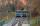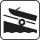# Ladder slope

What is the slope of a ladder 6.2 m long and 5.12 m in height.

Result

A =  55.67 °

#### Solution:Try calculation via our triangle calculator.

Leave us a comment of example and its solution (i.e. if it is still somewhat unclear...):

Showing 0 comments:Be the first to comment!#### To solve this example are needed these knowledge from mathematics:

See also our right triangle calculator. See also our trigonometric triangle calculator. Try conversion angle units angle degrees, minutes, seconds, radians, grads.

## Next similar examples:

1. Right triangleLadder 16 feet reaches up 14 feet on a house wall. The 90-degree angle at the base of the house and wall. What are the other two angles or the length of the leg of the yard?
2. Isosceles triangleWhat are the angles of an isosceles triangle ABC if its base is long a=5 m and has an arm b=4 m.
3. BuildingThe building I focused at an angle 30°. When I moved 5 m building I focused at an angle 45°. What is the height of the building?
4. 30-60-90The longer leg of a 30°-60°-90° triangle measures 5. What is the length of the shorter leg?
5. Theorem proveWe want to prove the sentense: If the natural number n is divisible by six, then n is divisible by three. From what assumption we started?
6. CablecarFunicular on Petrin (Prague) was 408 meters long and overcomes the difference 106 meters in altitude. Calculate the angle of climb.
7. Cable car 2Cable car rises at an angle 41° and connects the upper and lower station with an altitude difference of 1175 m. How long is the track of cable car?
8. Cable carCable car rises at an angle 45° and connects the upper and lower station with an altitude difference of 744 m. How long is "endless" tow rope?
9. MapleMaple peak is visible from a distance 3 m from the trunk from a height of 1.8 m at angle 62°. Determine the height of the maple.
10. Height 2Calculate the height of the equilateral triangle with side 38.
11. An angleAn angle x is opposite side AB which is 10, and side AC is 15 which is hypotenuse side in triangle ABC. Calculate angle x.
12. BoatA force of 300 kg (3000 N) is required to pull a boat up a ramp inclined at 14° with horizontal. How much does the boat weight?
13. Reference angleFind the reference angle of each angle:
14. Clock faceclock face is given. Numbers 10 and 5, and 3 and 8 are connected by straight lines. Calculate the size of their angles.
15. TrigonometryIs true equality? ?
16. SinesIn ▵ ABC, if sin(α)=0.5 and sin(β)=0.6 calculate sin(γ)
17. Centre of massThe vertices of triangle ABC are from the line p distances 3 cm, 4 cm and 8 cm. Calculate distance from the center of gravity of the triangle to line p.